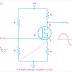The main difference between a voltage amplifier and a current amplifier is, voltage amplifier amplifies the voltage whereas the current amplifier amplifies the current. In other words, we can say the voltage amplifier provides higher voltage gain whereas the current amplifier provides higher current gain.

Let's go to know more differences between current amplifier and voltage amplifier in a detailed explanation.

You can better understand the difference if you have a clear knowledge of amplifier gain. The gain is basically the ratio of output and input. So the voltage gain is the ratio of output voltage and input voltage. Similarly, the current gain is the ratio of output current and input current.

So, in a current amplifier, the high current gain implies that a small change in the input current will cause a large change in the output current. Also, there a large amount of current will be flow in the output circuit when a small amount of current flowing in the input circuit.

Similarly, in a voltage amplifier, the high voltage gain implies that a small change in the input voltage will cause a large change in the output voltage. Also, a large amount of voltage will produce in the output when a small amount of voltage is applied to the input. Voltage amplifiers are mostly made up of voltage control semiconductor devices such as Field Effect Transistors or FETs whereas Current amplifiers are mostly made up of current-controlled semiconductor devices such as Bipolar Junction Transistors or BJTs.

Other key differences are,

1. Voltage amplifiers have very high impedance so they circulate low current. Generally, the voltage amplifiers provide high voltage gain and unity current gain. On the other hand, current amplifiers have low impedance so they circulate high current. So, the current amplifiers provide high current gain and unity voltage gain.

2. Voltage amplifiers are produced low power loss due to their high impedance and low current flow, on the other hand, current amplifiers are produced high power loss due to high current flow.

3. Voltage amplifiers are most suitable for audio signal amplification and driving loudspeakers whereas current amplifiers are most suitable for signal processing, pre-amplification, increase the power of transducer, etc.

4. Generally, the output and input voltage are almost equal for a current amplifier but input and output current differs. On the other hand, input and output current is almost equal for a voltage amplifier but input and output voltage differs.

5. Due to the high impedance, capacitance effect or miller effect occurs in voltage amplifiers but in current amplifiers, capacitance effect does not happen more.

6. Current amplifiers are very sensitive to changes in input current whereas voltage amplifiers are very sensitive to changes in voltage.

7. Leakage current mostly occurs in voltage amplifier circuits due to capacitance effects but in current amplifier leakage current happens in a very smaller amount than the normal current.

8. When voltage amplifiers are used with very high-frequency signals, noise or unwanted signals occurs but in the case of a current amplifier this happens in a very less amount.

Difference Between Voltage and Current Amplifier ExplainedReviewed by Author on 9/03/2021 Rating: 5